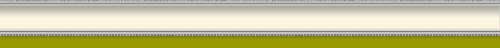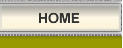# Compound Interest & Rule of 72

## Albert Einstien called it the most powerful thing he had ever witnessed.

Compound interest is a fact of life. The only real choice you have is whether you will have compound interest working for you in savings or against you in debt. But what exactly is compound interest? And what is the "Rule of 72"? [SPECIAL NOTE: Many thanks to the links at the upper right Elsewhere on the Web. Though the material is universally available and therefore public knowlege, recognition should still be issued for my "re-education"]

Compound Interest Defined - Let's start by illustrating what compound interest is all about. We all know interest is computed on an account balance (i.e. a savings account). But when interest is added to the account versus returning it immediately to the customer, the interest itself earns interest during the next time period for computing interest.

The time interval between the occasions at which interest is added to the account is called the Compounding Period. The interest rate (normally stated as an annual % in decimal format and sometimes called the nominal rate), together with the compounding period and the balance in the account, determines how much interest is added in each compounding period. The basic formula is this:

Interest rate (r) X Principle (P) or balance = the interest to be added (I)For example: If an Interest rate of 6% (r) were applied to a Principle (P) of \$10,000, the interest (I) would be \$600.

\$10000 X 6% = \$600

The compound Interest Equation is:     P = A (1 + r/n)^nt

where:

• P = the principal (current worth)
• A = the initial amount on deposit
• r = the interest rate (expressed as a fraction: ex: 6% = .06)
• n = the number of times per year that interest is compounded
• t = the number of years invested

NOTE: The above might be more easily understod thinking in terms of a Simplified Compound Interest . When interest is only compounded once per yer (n=1), the equation simplifies to: P = A (1 + r)^t

• P = \$10,000 X (1 + .06) X 1 year
• P = \$10,600

The following table shows the final principal (P), after t = 1 year, of an account initally with P = \$10000, at 6% interest rate, with the given compounding (n).

•      n                        P
• 1 (yearly)              \$ 10600.00
• 2 (semi-anually)     \$ 10609.00
• 4 (quarterly)          \$ 10613.64
• 12 (monthly)          \$ 10616.78
• 52 (weekly)           \$ 10618.00
• 365 (daily)            \$ 10618.31
• continuously          \$ 10618.37

As you can see, there is an advantage to compounding more frequently. If the balance, interest rate and length of the deposit all remain the same, more interest can be earned by increasing the compounding periods per year. With the information above and armed with the "Rule of 72" information below, you can start to understand why a \$100,000 home over a 30 year loan period can actually cost over \$300,000.

[Special note: When interest is compounded continually (in other words, when 'n' approaches infinity), the compound interest equation takes the form: P = A e rt where e is approximately 2.71828 (the exponential number).

The "Rule of 72" -  allows you to determine the number of years before your money doubles... whether in debt or investment. Here is how to do it:

Divide the number 72 by the percentage rate you are paying on your debt (or earning on your investment).

For example: You borrowed \$1,000 at 6% interest. 72 divided by 6 is 12. That makes 12 the number of years it would take for your debt to double to \$2,000 if you did not make any payments. Similarly, A savings account with \$500 deposited in it earning 4% interest. 72 divided by 4 is 18. It will take 18 years for your \$500 to double to \$1,000 if you don't make any deposits.

Now, consider compounding the compound interest or doubling the Rule of 72. See what happens each time your money doubles...

\$1 ... \$2 ... \$4 ... \$8 ... \$16 ... \$32 ... \$64 ... \$128 ...Consider how fast your debts can double with high interest rates, such as those charged on most credit card accounts. On the ther hand, think what happens cashing in a long term mutual fund. Compound interest is like a mushroom cloud... the longer it exists, the more powerful a force it becomes... working for you or against you.

Adding Fuel To The Fire - Now permit me to put it all into perspective while adding fuel to the fire. In the article Balance Transfer and Consolidation you will find a couple very interesting tables. One of these tables offers information on applying the minimum monthly payment on a credit card which is typically 2%-3% of the outstanding balance. See what happens when varying the minimum payments. The table is based upon a \$5000 balance and a 17% interest rate.

You will soon discover that though the minimum monthly payment is dramatically reduced with a lower % minimum payment, the tota interest paid and years to pay off become astronomical.  By reducing the minimum paymetn by 1/2, the years to payoff increases from 18 years to 80 years.  The interst paid  raises from \$4200 to over \$25000.

Now ask yourself "Is compound interest working for or against you?"